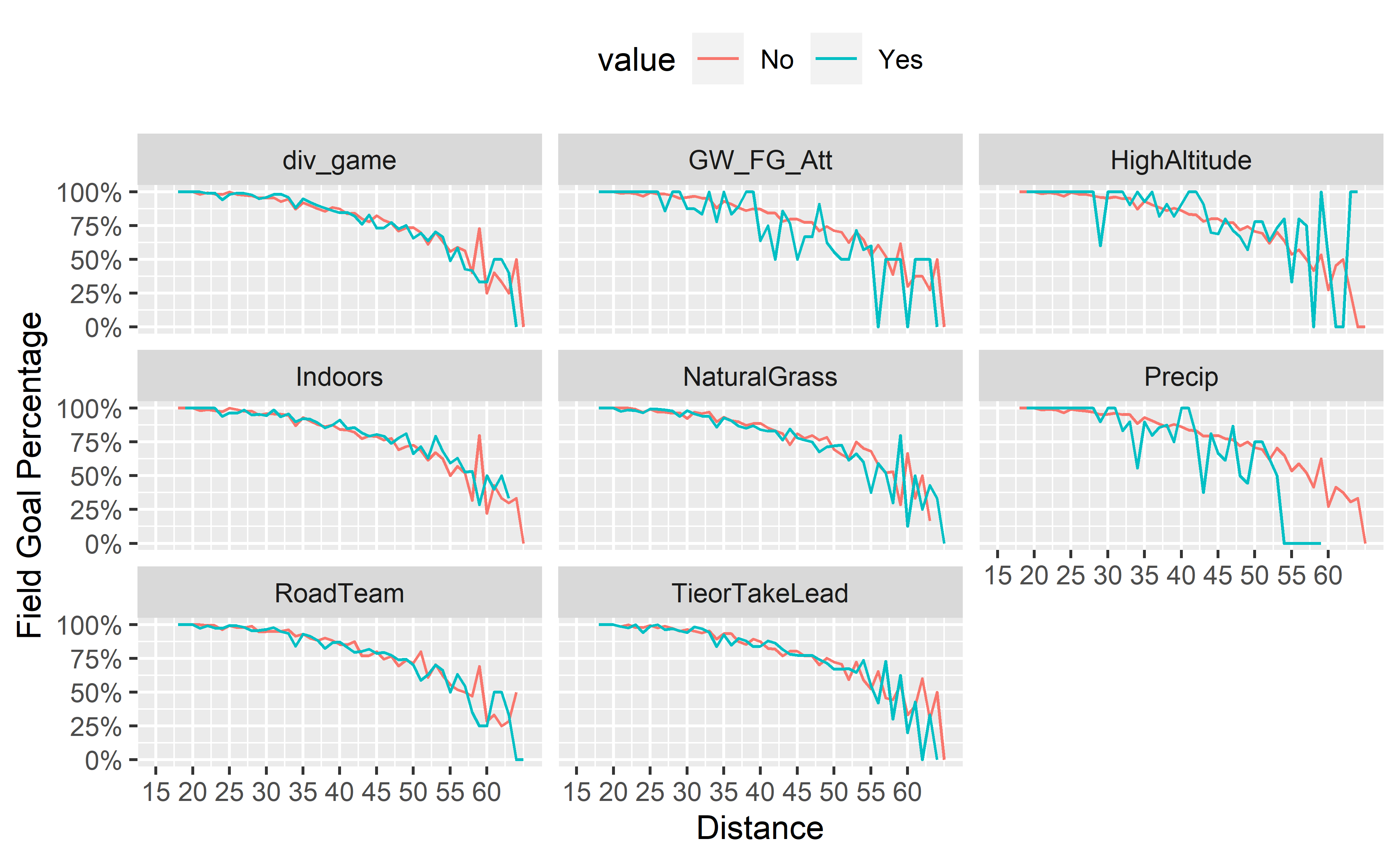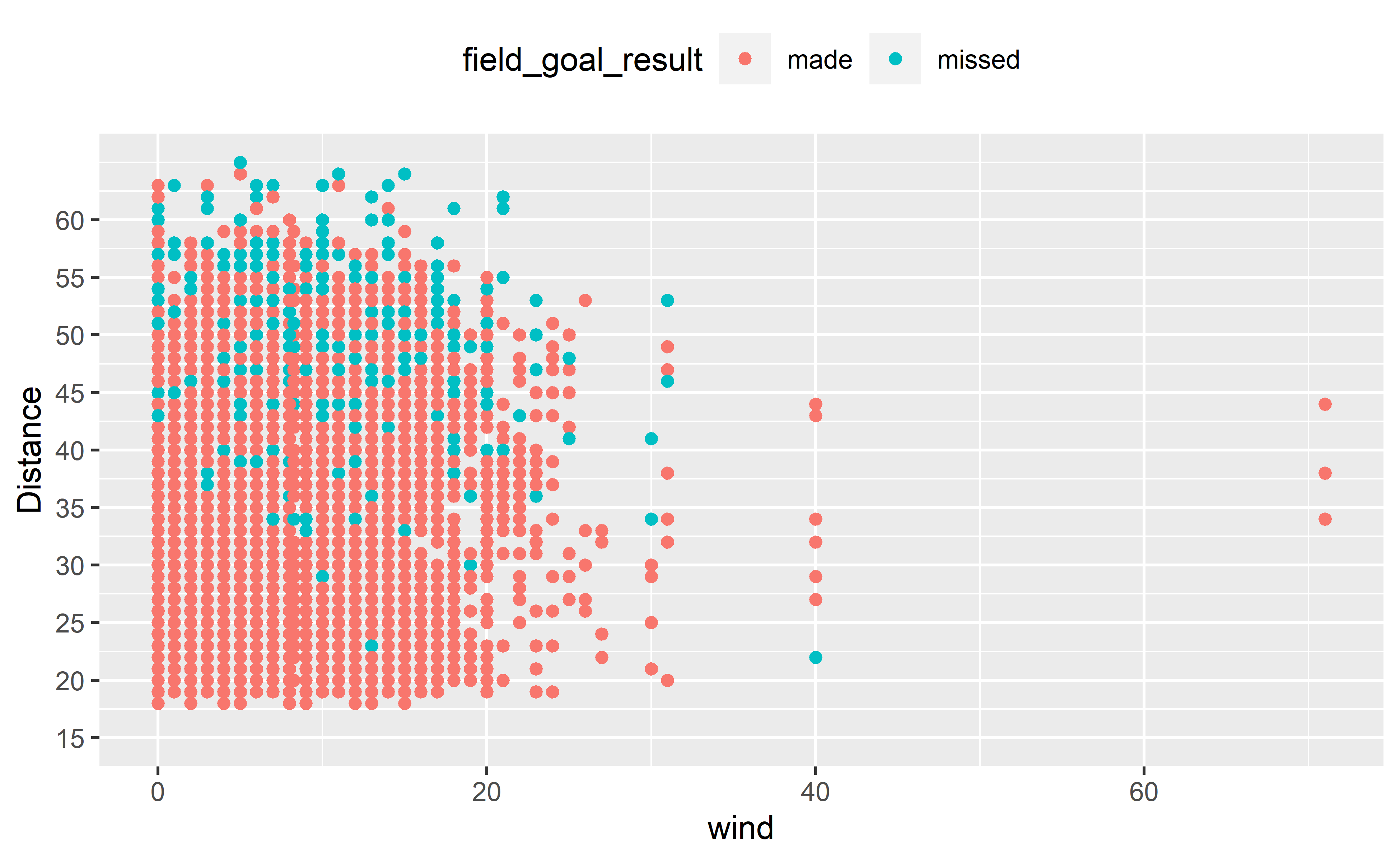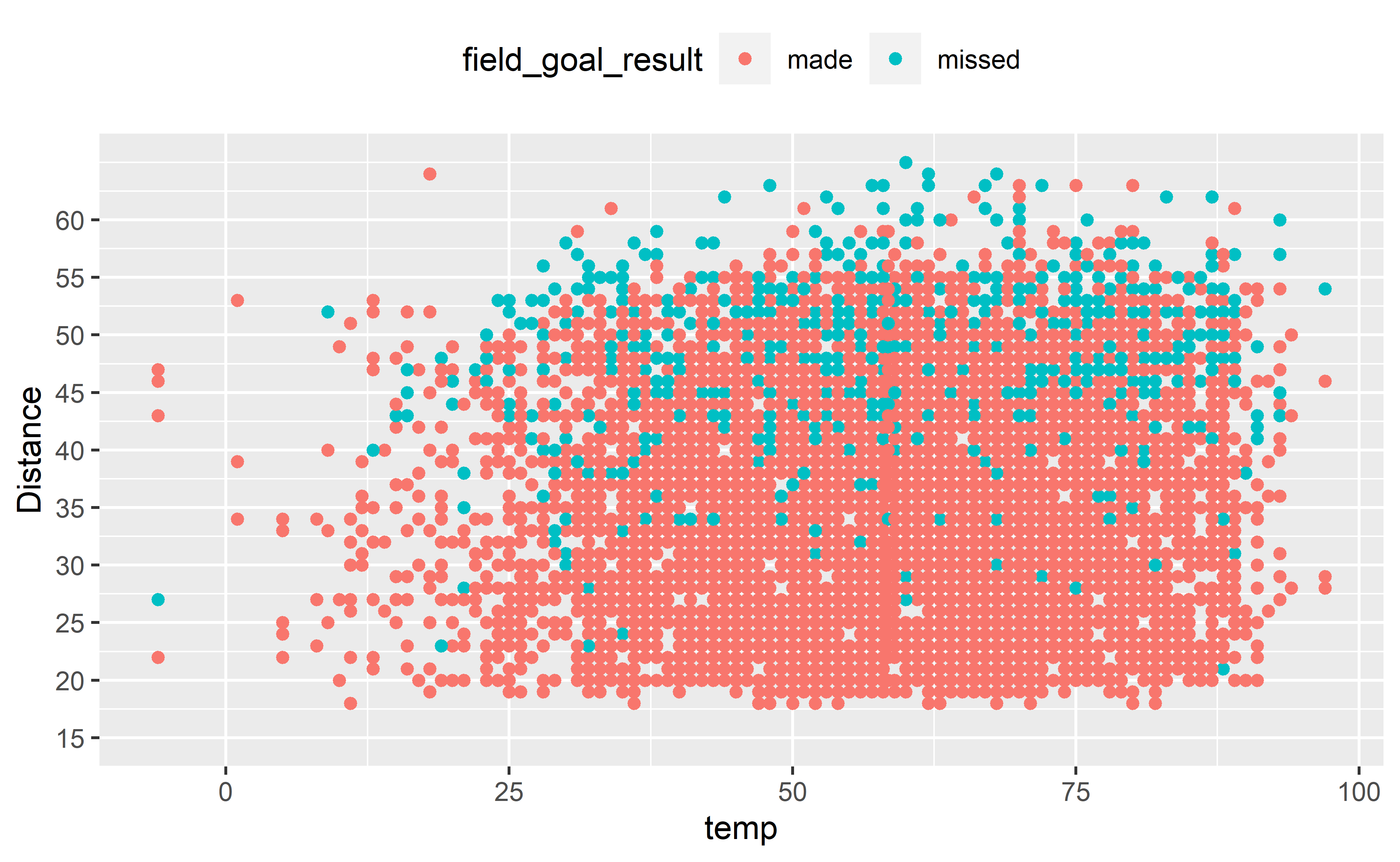Creating an Expected Field Goal Metric

Using nflfastR play-by-play data to measure kicker performance.

09-09-2020

Introduction

Placekickers are often an underappreciated portion of a football team. They receive plenty of criticism when important field goals are missed, and receive only a slight amount of praise when a big kick is made. A weak kicking game can be especially costly in close games and can turn wins into losses. By quantifying kicker performance, teams can try to prevent the kicker from being the reason for losing games that come down to a key field goal.

Field Goal Percentage is a common metric used to measure kicker performance, but it does not sufficiently account for varying levels of difficulty for each attempt. For example, a 19 yard attempt made in clear skies with no wind is much easier to make than a 50 yard attempt in the snow. To better account for the specific difficulty of each field goal attempt, I created an expected field goal model. This logistic regression model was developed using nflfastR’s play-by-play data from the 2009-2019 NFL seasons and determines the probability of a field goal being made, given the values of certain input variables. This expected field goal (xFG) metric is useful in measuring an individual kicker’s field goal performance, and could potentially be used to help coaches decide if their kicker has a strong probability of making a certain field goal attempt by factoring in a variety of conditions.

After reading in the play-by-play data, I created calculated columns as potential variables for the regression model. These calculated columns account for many attributes that may impact the result of a field goal attempt: indoors/outdoors, natural grass/artificial turf, precipitation, etc. Most of these fields contain binary Yes/No values, but “wind” and “temp” are continuous variables.

One issue I experienced was missing values for nflfastR’s “weather”, “temp”, and “wind” fields. Many of the missing values were a result of dome or closed roof stadiums, so those values were manually imputed with “No” for precipitation, 0 for wind, and 70 for temp. For missing values in outdoor stadiums, the impute.mean function replaced any missing values with the average value for that variable.

library(nflfastR)
library(tidyverse)
library(caret)
library(gt)

# Read in data from nflfastR
seasons <- 2009:2019
pbp <- map_df(seasons, function(x) {
url(
paste0("https://raw.githubusercontent.com/guga31bb/nflfastR-data/master/data/play_by_play_", x, ".rds")
)
)
})

# Create function to replace NA with mean value for temp and wind columns
impute.mean <- function(x) replace(x, is.na(x), mean(x, na.rm = TRUE))

# Create data frame with kicks from 2009-2019, including new factors for model
fg_data <- pbp %>%
filter(play_type == "field_goal", field_goal_result != "blocked") %>%
mutate(
div_game = factor(div_game, levels = c(0, 1), labels = c("No", "Yes")),
TieorTakeLead = as.factor(if_else(score_differential >= -3 & score_differential <= 0, "Yes", "No")),
GW_FG_Att = as.factor(if_else(score_differential >= -3 & score_differential <= 0 & qtr == 4 & as.numeric(gsub(":", "", (time))) < 100, "Yes", "No")),
RoadTeam = as.factor(if_else(posteam == away_team, "Yes", "No")),
Indoors = as.factor(if_else(roof == "closed" | roof == "dome", "Yes", "No")),
HighAltitude = as.factor(if_else(home_team == "DEN", "Yes", "No")),
NaturalGrass = as.factor(if_else(grepl("grass", surface, ignore.case = TRUE), "Yes", "No")),
Precip = as.factor(case_when(
grepl("closed", roof, ignore.case = TRUE) ~ "No",
grepl("dome", roof, ignore.case = TRUE) ~ "No",
grepl("snow", weather, ignore.case = TRUE) ~ "Yes",
grepl("showers", weather, ignore.case = TRUE) ~ "Yes",
grepl("0% Chance of Rain", weather, ignore.case = TRUE) ~ "No",
grepl("Cloudy, chance of rain increasing up to 75%", weather, ignore.case = TRUE) ~ "Yes",
grepl("Cloudy, chance of rain", weather, ignore.case = TRUE) ~ "No",
grepl("Zero Percent Chance of Rain", weather, ignore.case = TRUE) ~ "No",
grepl("Rain Chance 40", weather, ignore.case = TRUE) ~ "No",
grepl("30% Chance of Rain", weather, ignore.case = TRUE) ~ "No",
grepl("No chance of rain", weather, ignore.case = TRUE) ~ "No",
grepl("Cloudy, Humid, Chance of Rain", weather, ignore.case = TRUE) ~ "No",
grepl("rain", weather, ignore.case = TRUE) ~ "Yes",
TRUE ~ "No"
)),
wind = case_when(
is.na(wind) & roof != "outdoors" ~ 0,
is.na(wind) & roof == "outdoors" ~ impute.mean(wind),
!is.na(wind) ~ as.numeric(wind),
TRUE ~ 0
),
temp = case_when(
is.na(temp) & roof != "outdoors" ~ 70,
is.na(temp) & roof == "outdoors" ~ impute.mean(temp),
!is.na(temp) ~ as.numeric(temp),
TRUE ~ 0
)
)

Exploratory Variable Analysis

I created a series of line charts and scatter plots to take a look at which independent variables might have an impact on Field Goal Percentage (number of made kicks divided by all attempts). The line charts take a look at the impact of each categorical variable (combined with distance) on field goal percentage, while the scatter plots focus on continuous variables like wind and temperature.

# Line charts of field goal rate by distance - split by categorical variables
fg_data %>%
gather(metric, value, div_game:Precip) %>%
group_by(kick_distance, metric, value) %>%
summarize(
fg% = sum(field_goal_result == "made") / n(),
Attempts = n()
) %>%
arrange(kick_distance) %>%
ggplot(aes(x = kick_distance, y = fg%, group = value, color = value)) +
geom_line() +
scale_y_continuous(labels = scales::percent, name = "Field Goal Percentage") +
scale_x_continuous(breaks = seq(15, 60, by = 5), limits = c(15, 65), name = "Distance") +
theme(legend.position = "top") +
facet_wrap(~metric)# Scatter plots of field goal result by distance and wind/temp  - split by continuous variables
fg_data %>%
ggplot(aes(x = kick_distance, y = wind, group = field_goal_result, color = field_goal_result)) +
geom_point() +
scale_x_continuous(breaks = seq(15, 60, by = 5), limits = c(15, 65), name = "Distance") +
theme(legend.position = "top") +
coord_flip()# Scatter plots of field goal result by distance and wind/temp  - split by continuous variables
fg_data %>%
ggplot(aes(x = kick_distance, y = temp, group = field_goal_result, color = field_goal_result)) +
geom_point() +
scale_x_continuous(breaks = seq(15, 60, by = 5), limits = c(15, 65), name = "Distance") +
theme(legend.position = "top") +
coord_flip()For the categorical variables, the line charts show some larger variances in Field Goal Percentage based on precipitation (Precip), field surface (NaturalGrass), and if the kick was a game-winning attempt (GW_FG_Att). The scatter plots show some potential variability in the field goal result at higher wind speeds and lower temperatures.

Creating the Expected Field Goal (xFG) Model

To create the xFG model, the data was split into train (80%) and test (20%) subsets. The train subset was used to create the model, while the test subset was used to evaluate the performance of the xFG model. First, the logistic regression model was run using all variables, then a second model was created using only the statistically significant variables. The second model (xFG_model) was then used to predict the outcome of field goal attempts from the test subset. If the predicted “made” value was greater than 0.5 (50%), then the model predicted the field goal attempt was successful.

# Create Train and Test splits (80/20)
set.seed(123)
train <- fg_data %>% sample_frac(.8)
test <- setdiff(fg_data, train)

# Create Logistic Regression Model using all variables
kick_distance + div_game + NaturalGrass + temp + wind + TieorTakeLead + GW_FG_Att + RoadTeam + Indoors + Precip,
family = binomial(logit),
data = train
)
summary(xFG_model_all)

# Final xField Goal Model. Includes significant variables only
kick_distance + GW_FG_Att + wind + Precip,
family = binomial(logit),
data = train
)
summary(xFG_model)

# Predict using test set. create confusion matrix
predictions <- predict(xFG_model, test)
confusionMatrix(as.factor(if_else(predictions >= .5, 1, 0)), as.factor(test\$made))

# Predict xFG using model
xFG <- predict(xFG_model, fg_data, type = "response")
pred_fg_data <- data.frame(fg_data, xFG)

The xFG model was able to predict the field goal result with 86% accuracy. The model was much better at predicting if a field goal would be made (88%) than if the field goal would be missed (49%). For predictive purposes, this model does not provide a significant lift in accuracy over the 86% field goal percentage of our test sample. To improve on the predictive power of the xFG model, more detailed weather data could be pulled into the model to get the exact weather measurements at time of the field goal attempt.

xFG and FGOE

However, the xFG metric can still be useful in identifying kickers that converted more (or less) field goal attempts than expected. The chart below shows the top 10 kickers in Field Goals Over Expected (FGOE) for the 2019 Season:

# Field Goals over Expected Chart
pred_fg_data %>%
mutate(season = substr(game_id, 1, 4)) %>%
filter(season == 2019) %>%
group_by(kicker_player_name, posteam) %>%
summarize(
) %>%
arrange(-FGOE) %>%
ungroup() %>%
slice(1:10) %>%
mutate(Rank = paste0(row_number())) %>%
gt() %>%
tab_header(title = "Field Goals Over Expected - 2019 NFL Season") %>%
cols_move_to_start(columns = vars(Rank)) %>%
cols_label(
kicker_player_name = "Kicker",
posteam = "Team",
) %>%
fmt_number(columns = vars(xFG, FGOE), decimals = 1) %>%
cols_align(align = "center", columns = vars(Rank, kicker_player_name, posteam, xFG, ActualFGMade, FGOE)) %>%
tab_style(style = cell_text(size = "large"), locations = cells_title(groups = "title")) %>%
tab_style(style = cell_text(align = "center", size = "medium"), locations = cells_body()) %>%
tab_source_note(source_note = "") %>%
text_transform(
locations = cells_body(vars(posteam)),
fn = function(x) web_image(url = paste0("https://a.espncdn.com/i/teamlogos/nfl/500/", x, ".png"))
) %>%
data_color(columns = vars(FGOE), colors = "grey90", autocolor_text = FALSE) %>%
cols_width(vars(posteam) ~ px(45))
Field Goals Over Expected - 2019 NFL Season
Rank Kicker Team xFG FG Made FGOE
1 J.Tucker26.1 30 3.9
2 J.Lambo29.6 33 3.4
3 H.Butker33.5 36 2.5
4 K.Forbath8.3 10 1.7
5 C.Boswell27.3 29 1.7
6 D.Bailey28.5 30 1.5
7 M.Crosby20.6 22 1.4
8 R.Bullock25.9 27 1.1
9 B.McManus26.9 28 1.1
10 M.Prater25.0 26 1.0

Unsurprisingly, All-Pro Justin Tucker led all kickers in FGOE during the 2019 season. Interestingly, Kai Forbath only kicked in 4 games in 2019, but performed well enough in those opportunities to rank 4th among all kickers in FGOE.

Conclusion

The xFG metric is a useful tool to measure kicker performance because it accounts for the difficulty of each kick, including factors for distance, weather, and game situation. A kicker’s xFG can be compared to the actual number of field goals made to create FGOE. FGOE can help identify a kicker’s impact by rewarding kickers that make difficult field goal attempts, and penalizing those that miss attempts with a high probability of being made.

Corrections

If you see mistakes or want to suggest changes, please create an issue on the source repository.

Reuse

Text and figures are licensed under Creative Commons Attribution CC BY-NC 4.0. Source code is available at https://github.com/mrcaseb/open-source-football, unless otherwise noted. The figures that have been reused from other sources don't fall under this license and can be recognized by a note in their caption: "Figure from ...".